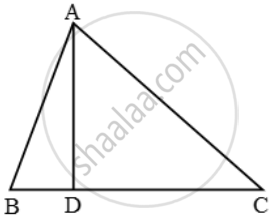# In figure, ∠BAC = 90º and segment AD ⊥ BC. Prove that AD^2 = BD × DC. - Mathematics

Sum

In figure, ∠BAC = 90º and segment AD ⊥ BC. Prove that AD2 = BD × DC.

#### Solution

In ∆ABD and ∆ACD, we haveTherefore, by AA-criterion of similarity, we have

∆DBA ~ ∆DAC

[ \therefore \ \ \angle D\leftrightarrow \ \angle D,\ \angleDBA\leftrightarrow \ \angle DAC\ and\ \angle BAD\leftrightarrow \ \angleDCA \]

\Rightarrow \frac{DB}{DA}=\frac{DA}{DC}

\Rightarrow \frac{BD}{AD}=\frac{AD}{DC}

AD^2 = BD × DC

Concept: Similarity
Is there an error in this question or solution?